# 多层感知机

## 隐藏层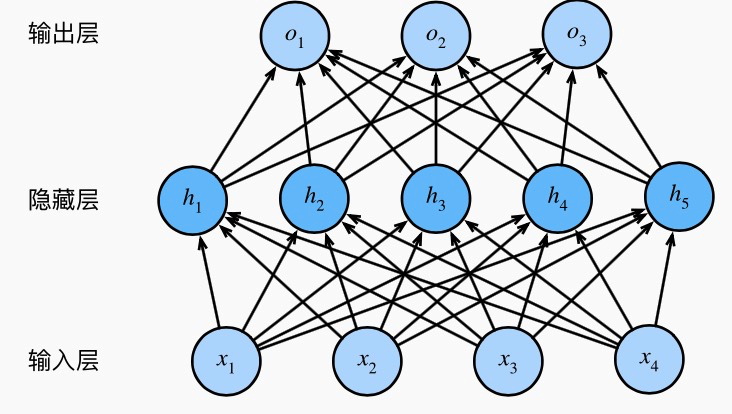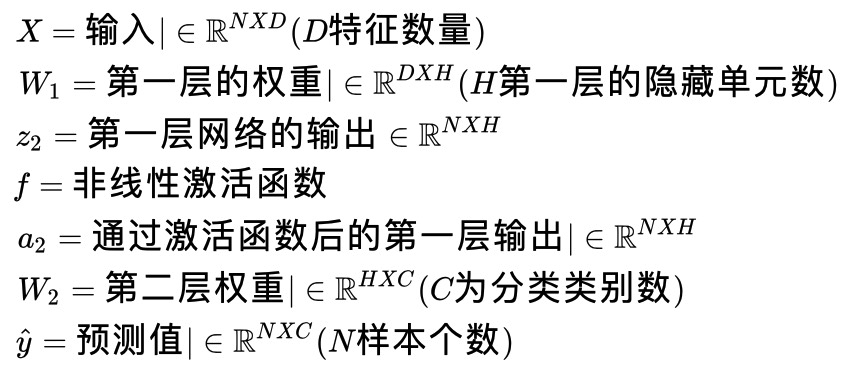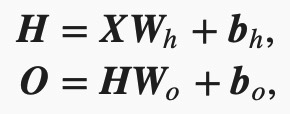也就是将隐藏层的输出直接作为输出层的输入。如果将以上两个式子联立起来，可以得到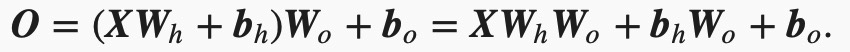从联立后的式子可以看出，虽然神经网络引入了隐藏层，却依然等价于一个单层神经网络：其中输出层权重参数为WhW0,偏差参数为bhW0+b。难发现，即便再添加更多的隐藏层，以上设计依然只能与仅含输出层的单层神经网络等价。

## 激活函数

### ReLU函数

ReLU（rectified linear unit）函数提供了一个很简单的非线性变换。给定元素xx，该函数定义为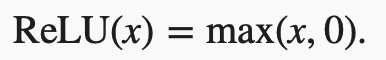%matplotlib inline
import d2lzh as d2l

def xyplot(x_vals, y_vals, name):
d2l.set_figsize(figsize=(5, 2.5))
d2l.plt.plot(x_vals.asnumpy(), y_vals.asnumpy())
d2l.plt.xlabel('x')
d2l.plt.ylabel(name + '(x)')

x = nd.arange(-8.0, 8.0, 0.1)
y = x.relu()
xyplot(x, y, 'relu')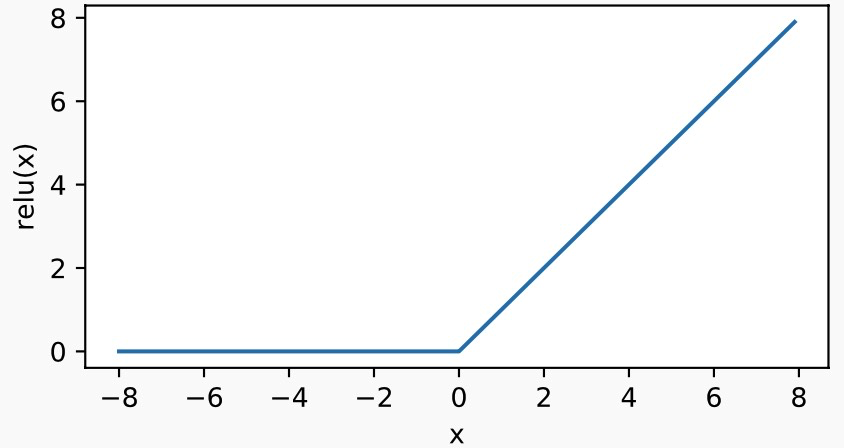y.backward()
xyplot(x, x.grad, 'grad of relu')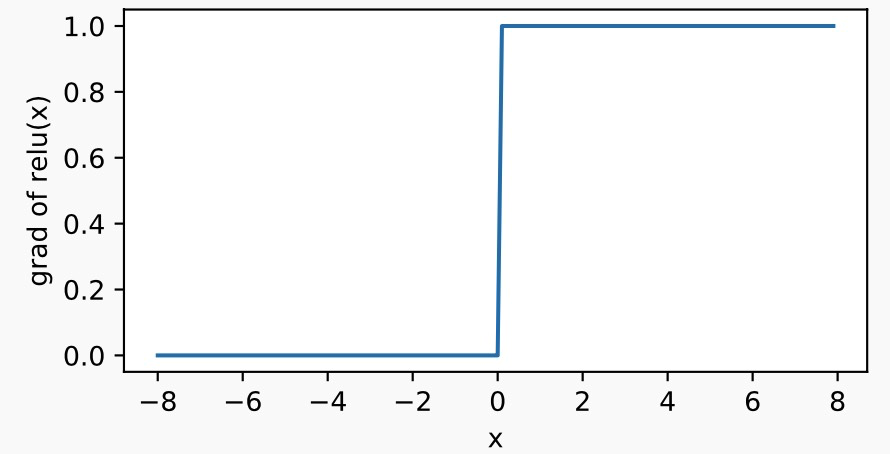### sigmoid函数

sigmoid函数可以将元素的值变换到0和1之间：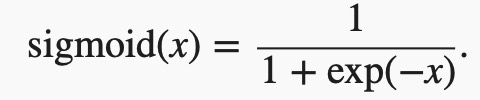sigmoid函数在早期的神经网络中较为普遍，但它目前逐渐被更简单的ReLU函数取代。在后面“循环神经网络”一章中我们会介绍如何利用它值域在0到1之间这一特性来控制信息在神经网络中的流动。下面绘制了sigmoid函数。当输入接近0时，sigmoid函数接近线性变换。

with autograd.record():
y = x.sigmoid()
xyplot(x, y, 'sigmoid')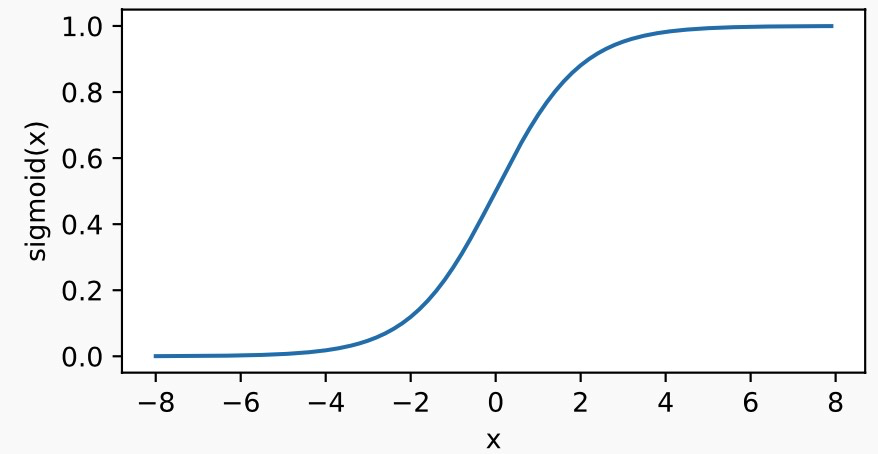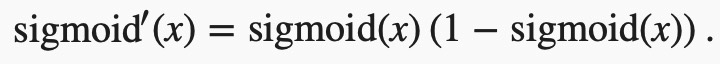y.backward()
xyplot(x, x.grad, 'grad of sigmoid')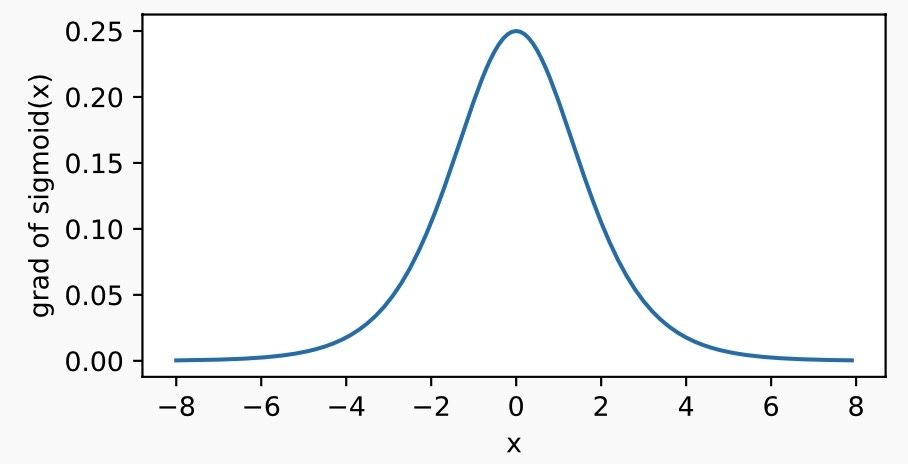### tanh函数

tanh（双曲正切）函数可以将元素的值变换到-1和1之间：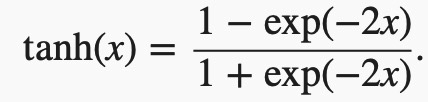with autograd.record():
y = x.tanh()
xyplot(x, y, 'tanh')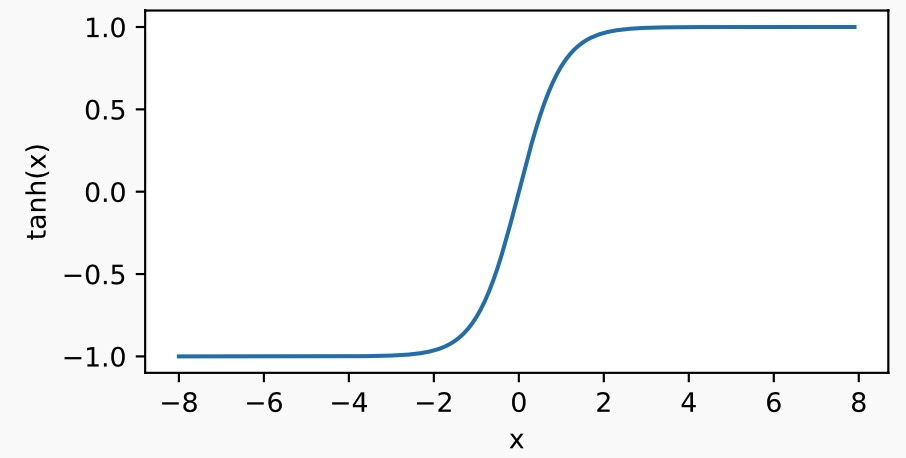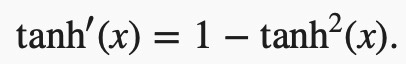y.backward()
xyplot(x, x.grad, 'grad of tanh')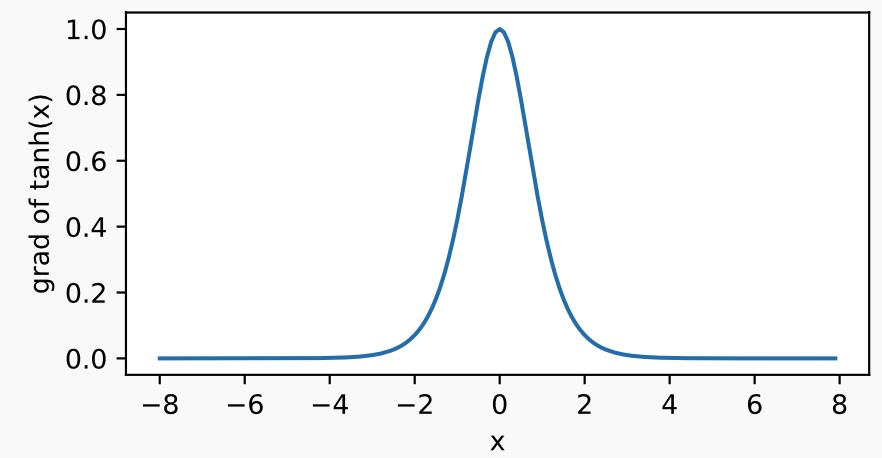## 多层感知机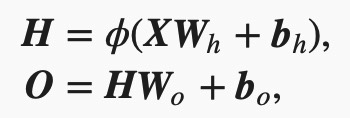## 代码实现

#!/usr/bin/env python
# coding: utf-8

# In:

get_ipython().run_line_magic('matplotlib', 'inline')
import d2lzh as d2l
from mxnet import nd
from mxnet.gluon import loss as gloss

# In:

#读取数据
batch_size = 256

# In:

#定义模型参数
#Fashion-MNIST数据集中图像形状为28×28，类别数为10。我们使用长度为28×28=784的向量表示每一张图像。
#因此，输入个数为784，输出个数为10。实验中，我们设超参数隐藏单元个数为256。
num_inputs, num_outputs, num_hiddens = 784, 10, 256

W1 = nd.random.normal(scale=0.01, shape=(num_inputs, num_hiddens))
b1 = nd.zeros(num_hiddens)
W2 = nd.random.normal(scale=0.01, shape=(num_hiddens, num_outputs))
b2 = nd.zeros(num_outputs)
params = [W1, b1, W2, b2]

for param in params:

# In:

#定义激活函数
def relu(X):
return nd.maximum(X, 0)

# In:

#定义模型
def net(X):
X = X.reshape((-1, num_inputs))
H = relu(nd.dot(X, W1) + b1)
return nd.dot(H, W2) + b2

# In:

#定义损失函数
loss = gloss.SoftmaxCrossEntropyLoss()

# In:

#训练模型
num_epochs, lr = 5, 0.5
d2l.train_ch3(net, train_iter, test_iter, loss, num_epochs, batch_size,
params, lr)

posted @ 2020-02-13 10:35  Jaww  阅读(...)  评论(...编辑  收藏